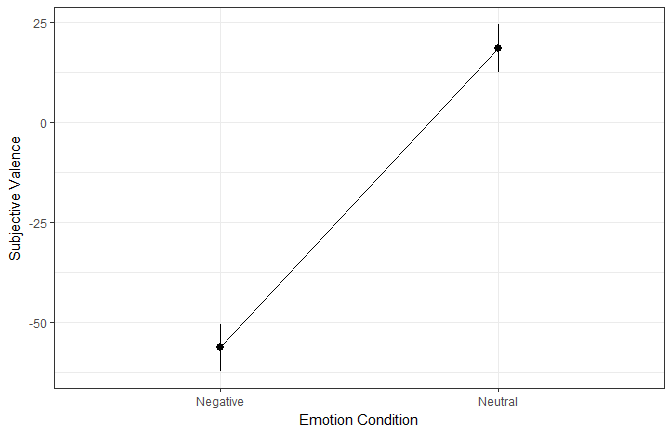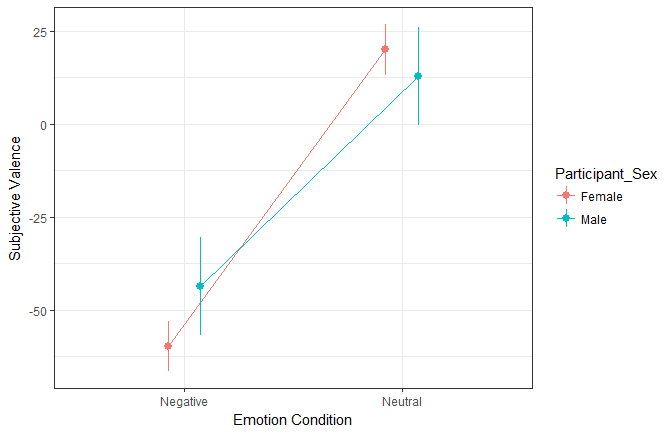01 May 2018 - Written by Dominique Makowski

# Don’t do it

Ha! Got ya! Trying to run some old school ANOVAs hum? I’ll show you even better!

There is now a tremendous amount of data showing the inadequacy of ANOVAs as a statistical procedure (Camilli, 1987; Levy, 1978; Vasey, 1987; Chang, 2009). Instead, many papers suggest moving toward the mixed-modelling framework (Kristensen, 2004; Jaeger, 2008), which was shown to be more flexible, accurate, powerful and suited for psychological data.

Using this framework, we will see how we can very simply answer our questions with R and the psycho package.

# The Emotion Dataset

Let’s take the example dataset included in the `psycho` package.

``````library(psycho)
library(tidyverse)

df <- psycho::emotion %>%
select(Participant_ID,
Participant_Sex,
Emotion_Condition,
Subjective_Valence,
Recall)

summary(df)
``````
`````` Participant_ID Participant_Sex Emotion_Condition Subjective_Valence
10S    : 48    Female:720      Negative:456      Min.   :-100.000
11S    : 48    Male  :192      Neutral :456      1st Qu.: -65.104
12S    : 48                                      Median :  -2.604
13S    : 48                                      Mean   : -18.900
14S    : 48                                      3rd Qu.:   7.000
15S    : 48                                      Max.   : 100.000
(Other):624
Recall
Mode :logical
FALSE:600
TRUE :312
``````

Our dataframe (called `df`) contains data from several participants, exposed to neutral and negative pictures (the `Emotion_Condition` column). Each row corresponds to a single trial. As there were 48 trials per participants, there are 48 rows by participant. During each trial, the participant had to rate its emotional valence (`Subjective_Valence`: positive - negative) experienced during the picture presentation. Moreover, 20min after this emotional rating task, the participant was asked to freely recall all the pictures he remembered.

Our dataframe contains, for each trial, 5 variables: the name of the participant (`Participant_ID`), its sex (`Participant_Sex`), the emotion condition (`Emotion_Condition`), the valence rating (`Subjective_Valence`) and whether the participant recalled the picture (`Recall`).

# The effect of Emotion

Does the emotion condition modulate the subjective valence? How to answer?

Whith a repeated measures ANOVA of course!

Let’s run it:

``````summary(aov(Subjective_Valence ~ Emotion_Condition + Error(Participant_ID/Emotion_Condition), data=df))
``````
``````Error: Participant_ID
Df Sum Sq Mean Sq F value Pr(>F)
Residuals 18 115474    6415

Error: Participant_ID:Emotion_Condition
Df  Sum Sq Mean Sq F value   Pr(>F)
Emotion_Condition  1 1278417 1278417   245.9 6.11e-12 ***
Residuals         18   93573    5198
---
Signif. codes:  0 '***' 0.001 '**' 0.01 '*' 0.05 '.' 0.1 ' ' 1

Error: Within
Df Sum Sq Mean Sq F value Pr(>F)
Residuals 874 935646    1070
``````

Wow, we found that there is a significant effect of the emotional condition on valence ratings. We might have Science material here.

As you know, an ANOVA is pretty much a condensed linear model where the predictors are factors. Therefore, we can run an ANOVA on a linear mixed model (which includes the “error” term, or random effect).

``````library(lmerTest)
fit <- lmer(Subjective_Valence ~ Emotion_Condition + (1|Participant_ID), data=df)
anova(fit)
``````
``````Type III Analysis of Variance Table with Satterthwaite's method
Sum Sq Mean Sq NumDF DenDF F value    Pr(>F)
Emotion_Condition 1278417 1278417     1   892    1108 < 2.2e-16 ***
---
Signif. codes:  0 '***' 0.001 '**' 0.01 '*' 0.05 '.' 0.1 ' ' 1
``````

As you can see, the results are, for the important bits (the sum of squares, mean square and p value), very close to those of the traditional approach.

Note that the `psycho` package, through the `analyze` function, also allows to display the interpretation of the underlying model itself with the following:

``````results <- analyze(fit)
print(results)
``````
``````The overall model predicting Subjective_Valence (formula = Subjective_Valence ~ Emotion_Condition + (1 | Participant_ID)) successfully converged and explained 56.73% of the variance of the endogen (the conditional R2). The variance explained by the fixed effects was of 52.62% (the marginal R2) and the one explained by the random effects of 4.11%. The model's intercept is at -56.34 (SE = 2.88, 95% CI [-62.07, -50.61]). Within this model:
- The effect of Emotion_ConditionNeutral is  significant (beta = 74.88, SE = 2.25, 95% CI [70.47, 79.29], t(892.00) = 33.29, p < .001***) and can be considered as medium (std. beta = 0.73, std. SE = 0.022).
``````

# Post-hoc / Contrast Analysis

Then, we wou’d like to see how the levels are different. To do this, we have to run a “contrast” analysis, comparing the estimated means of each level.

``````# We have to provide the model (here called fit and the factors we want to contrast
results <- get_contrasts(fit, "Emotion_Condition")
print(results\$contrasts)
``````
Contrast Difference SE df t.ratio p.value
Negative - Neutral -74.88 2.25 892 -33.29 0

It appears that the negative condition yields a significantly lower valence (i.e., more negative) than the neutral (-74.88 points of difference). At this point, we usually also want to know the means of each conditions. However, we often do it by directly computing the means and SDs of our observed data. But that’s not the cleanest way, as our data might be unbalanced or biased.

The best way to do it is to estimate means based on the fitted model (marginal means). Those were automatically computed when running the `get_contrasts` function. We just have to extract them.

Emotion_Condition Mean SE df CI_lower CI_higher
Negative -56.34 2.88 25.04 -62.27 -50.41
Neutral 18.54 2.88 25.04 12.61 24.47

Finally, we can plot these means:

``````library(ggplot2)

ggplot(results\$means, aes(x=Emotion_Condition, y=Mean, group=1)) +
geom_line() +
geom_pointrange(aes(ymin=CI_lower, ymax=CI_higher)) +
ylab("Subjective Valence") +
xlab("Emotion Condition") +
theme_bw()
``````# Interaction

Let’s repeat the previous steps with adding the participant’s sex as a predictor.

``````fit <- lmer(Subjective_Valence ~ Emotion_Condition * Participant_Sex + (1|Participant_ID), data=emotion)
anova(fit)
``````
``````Type III Analysis of Variance Table with Satterthwaite's method
Sum Sq Mean Sq NumDF DenDF  F value
Emotion_Condition                 703963  703963     1   891 621.8068
Participant_Sex                      520     520     1    17   0.4593
Emotion_Condition:Participant_Sex  20496   20496     1   891  18.1041
Pr(>F)
Emotion_Condition                 < 2.2e-16 ***
Participant_Sex                      0.5071
Emotion_Condition:Participant_Sex 2.313e-05 ***
---
Signif. codes:  0 '***' 0.001 '**' 0.01 '*' 0.05 '.' 0.1 ' ' 1
``````

It seems that there is a significant main effect of the emotion condition, as well as an interaction with the participants’ sex. Let’s plot the estimated means.

``````estimated_means <- get_means(fit, "Emotion_Condition * Participant_Sex")
estimated_means
``````
Emotion_Condition Participant_Sex Mean SE df CI_lower CI_higher
Negative Female -59.73 3.28 23.3 -66.51 -52.95
Neutral Female 20.05 3.28 23.3 13.27 26.83
Negative Male -43.63 6.35 23.3 -56.76 -30.50
Neutral Male 12.89 6.35 23.3 -0.24 26.02
``````ggplot(estimated_means, aes(x=Emotion_Condition, y=Mean, color=Participant_Sex, group=Participant_Sex)) +
geom_line(position = position_dodge(.3)) +
geom_pointrange(aes(ymin=CI_lower, ymax=CI_higher),
position = position_dodge(.3)) +
ylab("Subjective Valence") +
xlab("Emotion Condition") +
theme_bw()
``````Let’s investigate the contrasts:

``````get_contrasts(fit, "Emotion_Condition * Participant_Sex")
``````
Contrast Difference SE df t.ratio p.value
Negative,Female - Neutral,Female -79.78 2.51 891.0 -31.81 0.00
Negative,Female - Negative,Male -16.10 7.15 23.3 -2.25 0.14
Negative,Female - Neutral,Male -72.62 7.15 23.3 -10.16 0.00
Neutral,Female - Negative,Male 63.67 7.15 23.3 8.91 0.00
Neutral,Female - Neutral,Male 7.15 7.15 23.3 1.00 0.75
Negative,Male - Neutral,Male -56.52 4.86 891.0 -11.64 0.00

It appears that the differences between men and women is not significant. However, by default, `get_contrasts` uses the Tukey method for p value adjustment. We can, with an exploratory mindset, turn off the p value correction (or choose other methods such as bonferonni, fdr and such).

``````get_contrasts(fit, "Emotion_Condition * Participant_Sex", adjust = "none")
``````
Contrast Difference SE df t.ratio p.value
Negative,Female - Neutral,Female -79.78 2.51 891.0 -31.81 0.00
Negative,Female - Negative,Male -16.10 7.15 23.3 -2.25 0.03
Negative,Female - Neutral,Male -72.62 7.15 23.3 -10.16 0.00
Neutral,Female - Negative,Male 63.67 7.15 23.3 8.91 0.00
Neutral,Female - Neutral,Male 7.15 7.15 23.3 1.00 0.33
Negative,Male - Neutral,Male -56.52 4.86 891.0 -11.64 0.00

Without correcting for multiple comparisons, we observe that men rate the negative pictures as significantly less negative than women.

# Note

This analysis is even simpler in the Bayesian framework. See this tutorial.

# Credits

This package helped you? Don’t forget to cite the various packages you used :)

You can cite `psycho` as follows:

• Makowski, (2018). The psycho Package: an Efficient and Publishing-Oriented Workflow for Psychological Science. Journal of Open Source Software, 3(22), 470. https://doi.org/10.21105/joss.00470